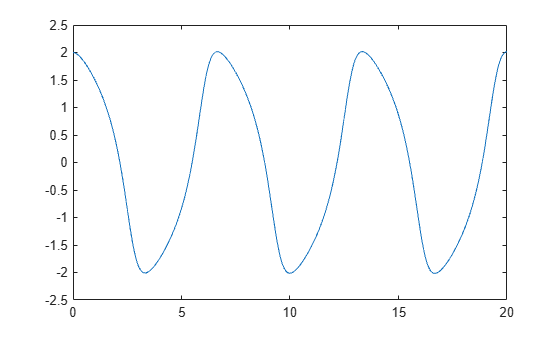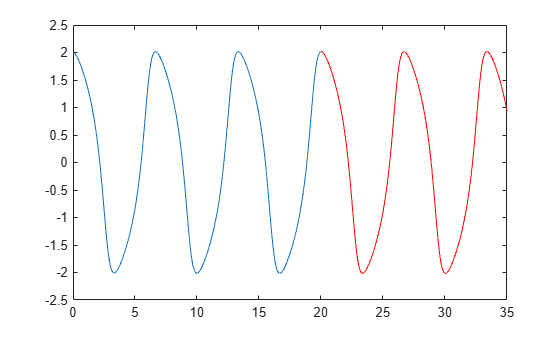# odextend

Extend solution to ODE

## Syntax

``solext = odextend(sol,odefun,tfinal)``
``solext = odextend(sol,[],tfinal)``
``solext = odextend(sol,odefun,tfinal,y0)``
``solext = odextend(sol,odefun,tfinal,y0,options)``

## Description

example

````solext = odextend(sol,odefun,tfinal)` extends the solution `sol` by integrating `odefun` from `sol.x(end)` to `tfinal`, using the same ODE solver that created `sol`. The function `odefun` can be different than the original function used to compute `sol`. The lower bound for the independent variable in `solext` is the same as in `sol`, that is, `sol.x(1)`. By default, `odextend` uses:The initial conditions `y = sol.y(:,end)` for the subsequent integration.The same integration properties and additional input arguments that the ODE solver originally used to compute `sol`. This information is stored in the solution structure `sol` and is later returned in `solext`. Unless you want to change these values, you do not need to pass them to `odextend`.```
````solext = odextend(sol,[],tfinal)` extends the solution to the same ODE function that was solved to obtain `sol`.```
````solext = odextend(sol,odefun,tfinal,y0)` specifies new initial conditions `y0` for the extended integration instead of using `sol.y(:,end)`.For the `ode15i` solver: `y0` must be an m-by-2 matrix containing column vectors of initial conditions for the solution components and their derivatives, ```y0 = [yinit ypinit]```.```
````solext = odextend(sol,odefun,tfinal,y0,options)` uses the integration options defined by `options`, which is an argument created using the `odeset` function. The specified options override the options that the ODE solver originally used to compute `sol`. You can optionally specify ```y0 = []``` to use default initial conditions.```

## Examples

collapse all

The van der Pol equation is a second order ODE

`${y}_{1}^{\prime \prime }-\mu \left(1-{y}_{1}^{2}\right){y}_{1}^{\prime }+{y}_{1}=0.$`

Solve the van der Pol equation with $\mu =1$ using `ode45`. The function `vdp1.m` ships with MATLAB® and encodes the equations. Specify a single output to return a structure containing information about the solution, such as the solver and evaluation points.

```tspan = [0 20]; y0 = [2 0]; sol = ode45(@vdp1,tspan,y0)```
```sol = struct with fields: solver: 'ode45' extdata: [1x1 struct] x: [0 1.0048e-04 6.0285e-04 0.0031 0.0157 0.0785 0.2844 0.5407 0.8788 1.4032 1.8905 2.3778 2.7795 3.1285 3.4093 3.6657 3.9275 4.2944 4.9013 5.3506 5.7998 6.2075 6.5387 6.7519 6.9652 7.2247 7.5719 8.1226 8.6122 9.1017 9.5054 9.8402 10.1157 ... ] y: [2x60 double] stats: [1x1 struct] idata: [1x1 struct] ```

Use `linspace` to generate 250 points in the interval `[0 20]`. Evaluate the solution at these points using `deval`.

```x = linspace(0,20,250); y = deval(sol,x);```

Plot the first component of the solution.

`plot(x,y(1,:))`Extend the solution to ${t}_{f}=35$ using `odextend` and add the result to the original plot.

```sol_new = odextend(sol,@vdp1,35); x = linspace(20,35,350); y = deval(sol_new,x); hold on plot(x,y(1,:),'r')```## Input Arguments

collapse all

Solution structure, specified as a structure returned by an ODE solver. When you call an ODE solver with a single output argument, it returns a solution structure.

Example: `sol = ode45(myode,tspan,y0)`

Data Types: `struct`

Function to solve, specified as a function handle. Use this input to extend the solution using a new or modified ODE function. To continue using the original ODE function used to create the solution structure `sol`, specify `odefun` as an empty input `[]`.

Data Types: `function_handle`

Final integration time, specified as a scalar.

Data Types: `single` | `double`

Initial conditions, specified as a scalar, vector, or matrix. By default `odextend` uses the initial conditions ```y = sol.y(:,end)``` to extend the integration. Use this input to specify new initial conditions for the extended integration.

For the `ode15i` solver: `y0` must be an m-by-2 matrix containing column vectors of initial conditions for the solution components and their derivatives, ```y0 = [yinit ypinit]```.

Data Types: `single` | `double`

Options structure. By default, `odextend` uses the same options and additional inputs as the ODE solver originally used to compute `sol`. Use this input to specify a new options structure that overrides the options used to create `sol`.

Use the `odeset` function to create or modify an ODE options structure.

Data Types: `struct`

## Output Arguments

collapse all

Extended solution, returned as a structure. Use this structure with the `deval` function to evaluate the solution at any point in the interval `[t0 tf]`. The `solext` structure array always includes these fields:

Structure FieldDescription

`sol.x`

Row vector of the steps chosen by the solver.

`sol.y`

Each column `sol.y(:,i)` contains the solution at time `sol.x(i)`.

`sol.solver`

Solver name.

Additionally, if you specify the `Events` option and events are detected, then `solext` also includes these fields:

Structure FieldDescription

`sol.xe`

Points when events occurred. `sol.xe(end)` contains the exact point of a terminal event, if any.

`sol.ye`

Solutions that correspond to events in `sol.xe`.

`sol.ie`

Indices into the vector returned by the function specified in the `Events` option. The values indicate which event the solver detected.

## Version History

Introduced before R2006a